Irrational Numbers

Chapter 1 Class 9 Number Systems
Concept wise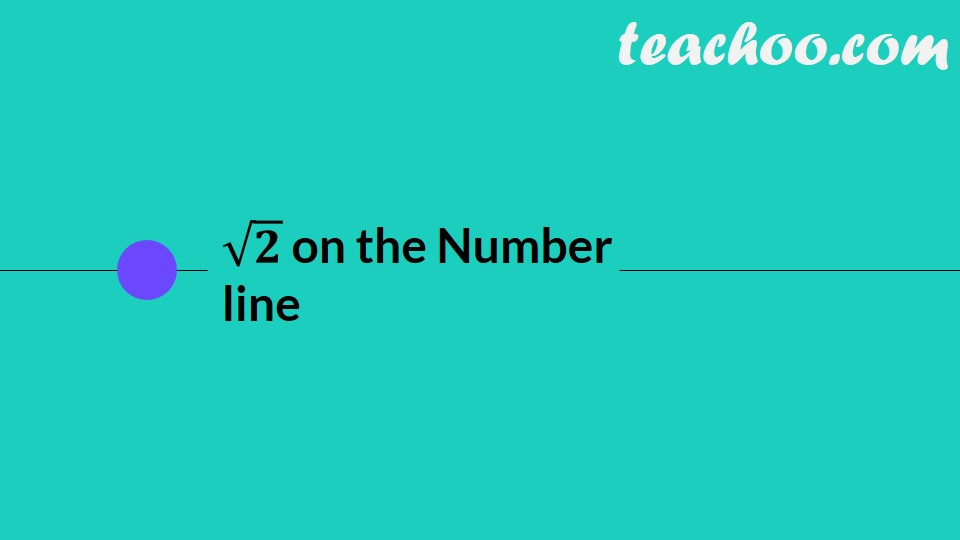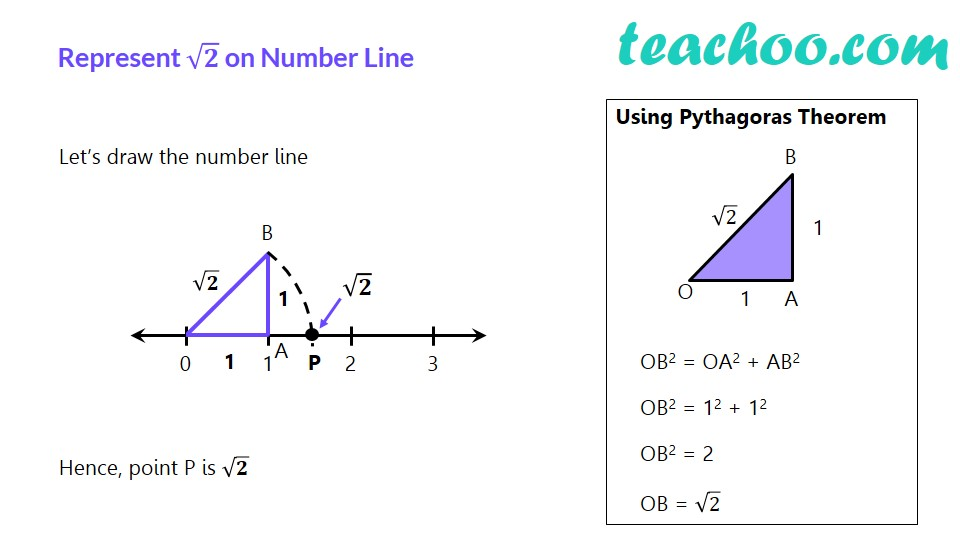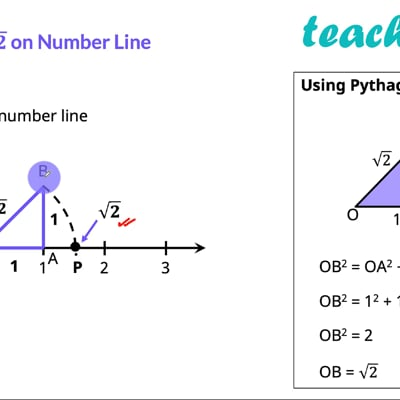This video is only available for Teachoo black users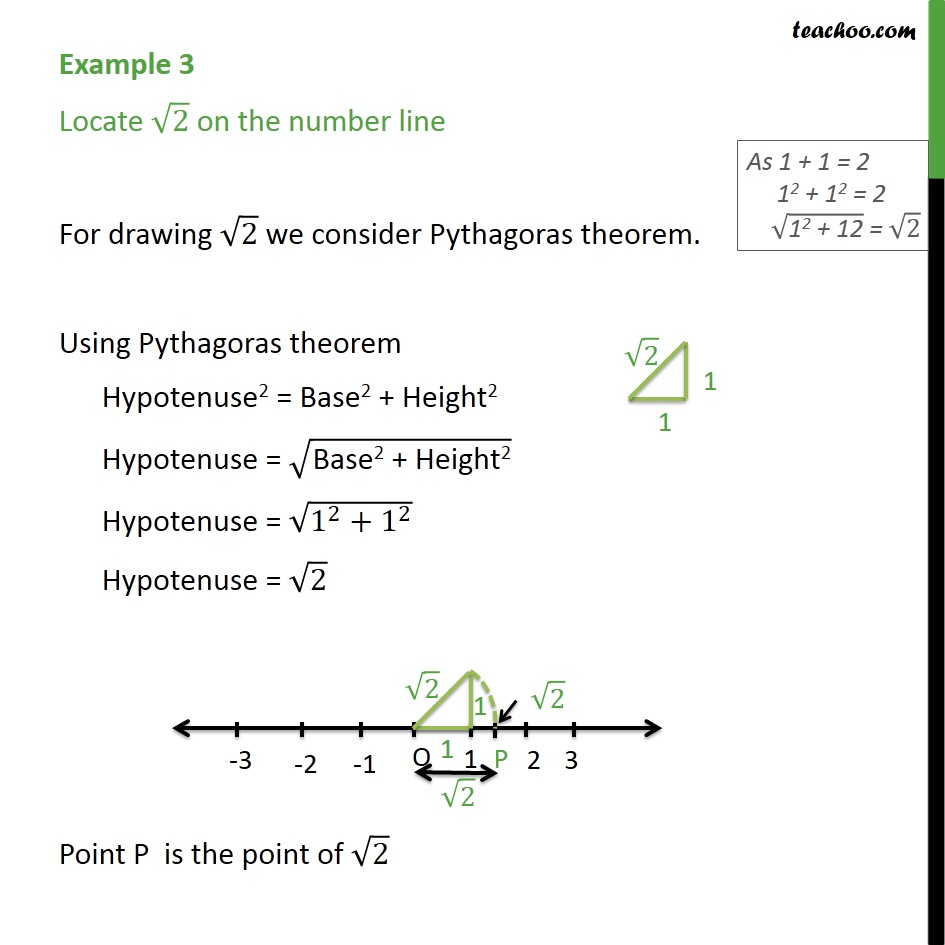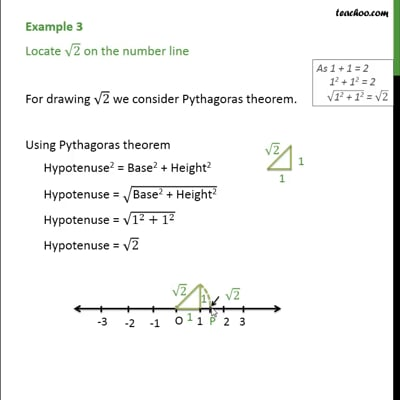This video is only available for Teachoo black users

Introducing your new favourite teacher - Teachoo Black, at only ₹83 per month

### Transcript

√𝟐 on the Number line Represent √𝟐 on Number Line Let’s draw the number line Hence, point P is √𝟐 Using Pythagoras Theorem OB2 = OA2 + AB2 OB2 = 12 + 12 OB2 = 2 OB = √2 Example 3 Locate 2 on the number line For drawing 2 we consider Pythagoras theorem. Using Pythagoras theorem Hypotenuse2 = Base2 + Height2 Hypotenuse = ("Base2 + Height2" ) Hypotenuse = (1^2+1^2 ) Hypotenuse = 2 Point P is the point of 2